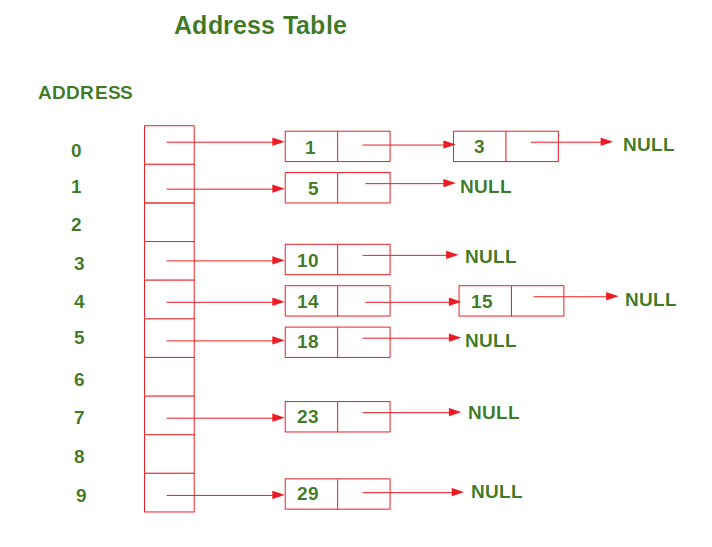# Address Calculation Sort using Hashing

• Difficulty Level : Easy
• Last Updated : 02 Sep, 2022

In this sorting algorithm, Hash Function f is used with the property of Order Preserving Function which states that ifHash Function:

```f(x) = floor( (x/maximum) * SIZE )
where maximum => maximum value in the array,
SIZE => size of the address table (10 in our case),
floor => floor function```

This algorithm uses an address table to store the values which is simply a list (or array) of Linked lists. The Hash function is applied to each value in the array to find its corresponding address in the address table. Then the values are inserted at their corresponding addresses in a sorted manner by comparing them to the values which are already present at that address.

Examples:

```Input : arr = [29, 23, 14, 5, 15, 10, 3, 18, 1]
Output:
After inserting all the values in the address table, the address table looks like this:

ADDRESS 0: 1 --> 3
ADDRESS 4: 14 --> 15

The below figure shows the representation of the address table for the example discussed above:After insertion, the values at each address in the address table are sorted. Hence we iterate through each address one by one and insert the values at that address in the input array. Below is the implementation of the above approach

Implementation:

## Python3

 `# Python3 code for implementation of``# Address Calculation Sorting using Hashing` `# Size of the address table (In this case 0-9)``SIZE ``=` `10` `class` `Node(``object``):` `    ``def` `__init__(``self``, data ``=` `None``):``        ``self``.data ``=` `data``        ``self``.nextNode ``=` `None` `class` `LinkedList(``object``):` `    ``def` `__init__(``self``):``        ``self``.head ``=` `None` `    ``# Insert values in such a way that the list remains sorted``    ``def` `insert(``self``, data):``        ``newNode ``=` `Node(data)` `        ``# If there is no node or new Node's value``        ``# is smaller than the first value in the list,` `        ``# Insert new Node in the first place``        ``if` `self``.head ``=``=` `None` `or` `data < ``self``.head.data:``            ``newNode.nextNode ``=` `self``.head``            ``self``.head ``=` `newNode` `        ``else``:``            ``current ``=` `self``.head``            ` `            ``# If the next node is null or its value``            ``# is greater than the new Node's value,` `            ``# Insert new Node in that place``            ``while` `current.nextNode !``=` `None` `\``                    ``and` `\``                    ``current.nextNode.data < data:``                ``current ``=` `current.nextNode` `            ``newNode.nextNode ``=` `current.nextNode``            ``current.nextNode ``=` `newNode``            ` `# This function sorts the given list``# using Address Calculation Sorting using Hashing``def` `addressCalculationSort(arr):` `    ``# Declare a list of Linked Lists of given SIZE``    ``listOfLinkedLists ``=` `[]``    ``for` `i ``in` `range``(SIZE):``        ``listOfLinkedLists.append(LinkedList())` `    ``# Calculate maximum value in the array``    ``maximum ``=` `max``(arr)` `    ``# Find the address of each value``    ``# in the address table``    ``# and insert it in that list``    ``for` `val ``in` `arr:``        ``address ``=` `hashFunction(val, maximum)``        ``listOfLinkedLists[address].insert(val)``    ` `    ``# Print the address table``    ``# after all the values have been inserted``    ``for` `i ``in` `range``(SIZE):``        ``current ``=` `listOfLinkedLists[i].head``        ``print``(``"ADDRESS "` `+` `str``(i), end ``=` `": "``)` `        ``while` `current !``=` `None``:``            ``print``(current.data, end ``=` `" "``)``            ``current ``=` `current.nextNode` `        ``print``()``    ` `    ``# Assign the sorted values to the input array``    ``index ``=` `0``    ``for` `i ``in` `range``(SIZE):``        ``current ``=` `listOfLinkedLists[i].head` `        ``while` `current !``=` `None``:``            ``arr[index] ``=` `current.data``            ``index ``+``=` `1``            ``current ``=` `current.nextNode``            `  `# This function returns the corresponding address``# of given value in the address table``def` `hashFunction(num, maximum):` `    ``# Scale the value such that address is between 0 to 9``    ``address ``=` `int``((num ``*` `1.0` `/` `maximum) ``*` `(SIZE``-``1``))``    ``return` `address` `# -------------------------------------------------------``# Driver code` `# giving the input address as follows``arr ``=` `[``29``, ``23``, ``14``, ``5``, ``15``, ``10``, ``3``, ``18``, ``1``]` `# Printing the Input array``print``(``"\nInput array: "` `+` `" "``.join([``str``(x) ``for` `x ``in` `arr]))` `# Performing address calculation sort``addressCalculationSort(arr)` `# printing the result sorted array``print``(``"\nSorted array: "` `+` `" "``.join([``str``(x) ``for` `x ``in` `arr]))`

Output

```Input array: 29 23 14 5 15 10 3 18 1
ADDRESS 0: 1 3
ADDRESS 4: 14 15
Time Complexity: The time complexity of this algorithm isin the best case. This happens when the values in the array are uniformly distributed within a specific range.
Whereas the worst-case time complexity is. This happens when most of the values occupy 1 or 2 addresses because then significant work is required to insert each value at its proper place.